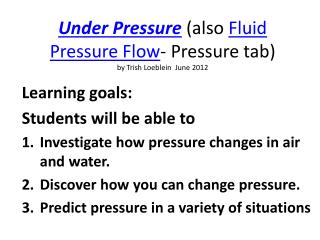DownloadDownload PresentationUnder Pressure (also Fluid Pressure Flow - Pressure tab ) by Trish Loeblein June 2012

# Under Pressure (also Fluid Pressure Flow - Pressure tab ) by Trish Loeblein June 2012

Download Presentation## Under Pressure (also Fluid Pressure Flow - Pressure tab ) by Trish Loeblein June 2012

- - - - - - - - - - - - - - - - - - - - - - - - - - - E N D - - - - - - - - - - - - - - - - - - - - - - - - - - -
##### Presentation Transcript

1. Under Pressure (also Fluid Pressure Flow- Pressure tab)by Trish Loeblein June 2012 Learning goals: Students will be able to Investigate how pressure changes in air and water. Discover how you can change pressure. Predict pressure in a variety of situations

2. 1. Order from lowest to highest pressure. • A<B<C • C<B<A • all are equal

3. 2. Look at the markers. Order from lowest to highest pressure. X A. Y<Z<X B. Y<X<Z C. Z<X<Y D. X<Z<Y E. two are equal Y Z

4. 3. What will happen to the pressure if more water is added? • increase • decrease • stay the same

5. 4. What will happen to the pressure if more water is added while the same amount is removed? • increase • decrease • stay the same

6. 5. What will happen to the pressure if the fluid were changed to honey? • increase • decrease • stay the same

7. 6. If the 250 kg mass was put on the water column, what will happen to the pressure? • increase • decrease • stay the same

8. 7. If the only change was to remove the air pressure , what will happen to the pressure? • increase by 101.3 kPa • decrease by 101.3 kPa • stay the same • Something else

9. 8. If the only change was to go to a place where the gravity was doubled, what will happen to the pressure? • Both pressures would double • Only the air pressure would double • The air pressure would double, and the water pressure would increase some • Something else

10. 9. How do the pressures at the two locations compare? • X>Y • Y>X • They are the same X Y